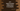# log function in C++ explanation with example# Introduction :

log() function in C++ is used to find out the base-e logarithm or natural logarithm of a number. It has different overloaded versions to take different types of arguments.

log() is defined in cmath header file and this header file is required to be imported at the top for using this function.

In this tutorial, we will learn how log() function can be used with examples.

## Definition of log() :

The log() method is defined as below (as per C++ 11 standard) :

``````double log (double x);
float log (float x);
long double log (long double x);
double log (T x); ``````

The last one if for integral type arguments. This overload actually cast the argument to a double before doing the calculation.

We will check all of these methods in this tutorial.

## Return value of log() :

If the argument is positive, it will return the natural logerithm value of the argument. If the argument is negative, it will cause one domain error. If the argument is zero, it may cause a pole error.

So, the best practice is to check if the number is greater than zero or not before using the log() function.

## Example :

Let me show you with an example of log() with different types of arguments :

``````#include <iostream>
#include <cmath>
using namespace std;

int main()
{
double a = 10.245;
float b = 12.3;
long double c = 14.623;
int d = 10;

cout << "log(a) : " << log(a) << endl;
cout << "log(b) : " << log(b) << endl;
cout << "log(c) : " << log(c) << endl;
cout << "log(d) : " << log(d) << endl;

cout << "log(1) : " << log(1) << endl;

return 0;
}``````

It will print the below output :

``````log(a) : 2.32679
log(b) : 2.5096
log(c) : 2.6826
log(d) : 2.30259
log(1) : 0``````